• 2022-03-12 14:58:03
"""
朴素贝叶斯算法案例
"""
import numpy as np
#准备数据
"""
加载数据集
:return: dataMatrix,labelList
"""
dataMatrix = [
["stop", "fuck", "you", "bitch", "garbage"],
["useless", "dog", "stupid", "worthless"],
["suck", "my", "dick", "bitch", "pig", "asshole"],
["son", "bitch", "hoocker", "happy"],
['my', 'dog', 'has', 'flea', 'problems', 'help', 'please'],
['my', 'dalmation', 'is', 'so', 'cute', 'I', 'love', 'him'],
['mr', 'licks', 'ate', 'my', 'steak', 'how', 'to', 'stop', 'him'],
]
labelList = [1, 1, 1, 1, 0, 0, 0]

return dataMatrix, labelList

#得到去重列表（词汇表）
def buildWordsSet(dataMatrix):
"""
创建单词集合
:param dataMatrix: 单词矩阵
:return:
"""
# 定义一个空set,因为set本身有去重的功能
wordsSet = set([])
# 遍历矩阵的每一行
for comment in dataMatrix:
# |表示取交集
wordsSet = wordsSet | set(comment)
return list(wordsSet)

#把dataMatrix转为one-hot
def getOneZeroVector(wordSet, comment):
"""
以wordSet这个训练集词频向量为依据构建comment的词频（0,1）向量
:param wordSet: 全集
:param comment: 单个评论的list
:return: 每个comment对应的(0,1)向量
"""
one_zero_vec =  * len(wordSet)
for word in comment:
if word in wordSet:
one_zero_vec[wordSet.index(word)] = 1
else:
print(" %s 没有收录" % word)
return one_zero_vec

#训练：
#计算bayes参数代码
def getBayesParams(one_zero_matrix, labelList):
"""
计算贝叶斯公式参数
:param one_zero_matrix: （0,1）词频矩阵
:param labelList: 每个评论的标签
:return: log（词频/好类总词数） ， log（词频/坏类总次数） ， 侮辱性评论占训练集总评论概率
"""
# 侮辱性评论概率
p_c1 = sum(labelList) / float(len(labelList))
# 训练集总词数
total_words_count = len(one_zero_matrix)
# 两种单词出现频率列表
p0List = np.ones(len(one_zero_matrix))
p1List = np.ones(len(one_zero_matrix))
# 计算两类词频
p0num = 1.0
p1num = 1.0

# 遍历所有测试集评论
for i in range(len(labelList)):
# 若该评论是侮辱性
if labelList[i] == 1:
p1List += one_zero_matrix[i]
p1num += sum(one_zero_matrix[i])
else:
p0List += one_zero_matrix[i]
p0num += sum(one_zero_matrix[i])
# 每个词词频列表/该类别词频 再取对数
p1vec = np.log(p1List / p1num)  # 已知是侮辱性评论情况下，每个词出现的概率
p0vec = np.log(p0List / p0num)  # 已知不是侮辱性评论情况下，每个词出现的概率

return p1vec, p0vec, p_c1

def classifyByBayes(p1vec, p0vec, p_c1, one_zero_vector):
"""
使用贝叶斯参数比较得出结果
:param p1vec:
:param p0vec:
:param p_c1:
:param one_zero_vector:
:return:
"""
# sum(one_zero_vector * p1vec) 对应元素相乘相加
# p_1 = sum(one_zero_vector * p1vec) + np.log(p_c1)
p_1 = sum(one_zero_vector * p1vec)
# p_0 = sum(one_zero_vector * p0vec) + np.log(1.0 - p_c1)
p_0 = sum(one_zero_vector * p0vec)
if p_1 > p_0:
return 1
else:
return 0python 机器学习
更多相关内容
• 主要介绍了Java实现的朴素贝叶斯算法,结合实例形式分析了基于java的朴素贝叶斯算法定义及样本数据训练操作相关使用技巧,需要的朋友可以参考下
• 朴素贝叶斯算法就是在牺牲一定准确率的情况下强制特征ｘ满足独立条件，求解P（x|y）就更为方便了 但基本上现实生活中，没有任何关系的两个特征几乎是不存在的，故朴素贝叶斯不适合那些关系密切的特征 from ...
• 本文实例讲述了朴素贝叶斯算法的python实现方法。分享给大家供大家参考。具体实现方法如下： 朴素贝叶斯算法优缺点 优点：在数据较少的情况下依然有效，可以处理多类别问题 缺点：对输入数据的准备方式敏感 适用数据...
• 《机器学习》算法实例-朴素贝叶斯算法-屏蔽社区留言板的侮辱言论 构建一个快速过滤器来屏蔽在线社区留言板上的侮辱性言论。如果某条留言使用了负面或者侮辱性的语言，那么就将该留言标识为内容不当。对此问题建立两...
• 大作业的任务是用朴素贝叶斯算法分析天气的和环境的好坏决定是否出门打网球。首先构建训练集；再实现分类算法，通过分类算法对训练数据集的各个特征属性分析，计算出各个特征属性的概率及每个特征属性划分对每个类别...大数据
• 本文实例讲述了Python实现的朴素贝叶斯算法。分享给大家供大家参考，具体如下： 代码主要参考机器学习实战那本书，发现最近老外的书确实比中国人写的好，由浅入深，代码通俗易懂，不多说上代码： #encoding:utf-8 '...
• 朴素贝叶斯算法案例 文章目录朴素贝叶斯算法案例朴素贝叶斯案例流程代码朴素贝叶斯算法总结 sklearn20类新闻分类 20个新闻组数据集包含20个主题的18000个新闻组帖子 朴素贝叶斯案例流程 1、加载20类新闻数据，并...

# 朴素贝叶斯算法案例

### 文章目录

• sklearn20类新闻分类
• 20个新闻组数据集包含20个主题的18000个新闻组帖子

## 朴素贝叶斯案例流程

1、加载20类新闻数据，并进行分割
2、生成文章特征词
3、朴素贝叶斯estimator流程进行预估

## 代码

from sklearn.datasets import load_iris, fetch_20newsgroups, load_boston
from sklearn.model_selection import train_test_split, GridSearchCV
from sklearn.neighbors import KNeighborsClassifier
from sklearn.preprocessing import StandardScaler
from sklearn.feature_extraction.text import TfidfVectorizer
from sklearn.naive_bayes import MultinomialNB
from sklearn.metrics import classification_report
from sklearn.feature_extraction import DictVectorizer
from sklearn.tree import DecisionTreeClassifier, export_graphviz
from sklearn.ensemble import RandomForestClassifier
import pandas as pd

# print("获取特征值")
# print(li.data)
# print("目标值")
# print(li.target)
# print(li.DESCR)

# 注意返回值, 训练集 train  x_train, y_train        测试集  test   x_test, y_test
# x_train, x_test, y_train, y_test = train_test_split(li.data, li.target, test_size=0.25)
#
# print("训练集特征值和目标值：", x_train, y_train)
# print("测试集特征值和目标值：", x_test, y_test)

# news = fetch_20newsgroups(subset='all')
#
# print(news.data)
# print(news.target)
#
#
# print("获取特征值")
# print(lb.data)
# print("目标值")
# print(lb.target)
# print(lb.DESCR)

def knncls():
"""
K-近邻预测用户签到位置
:return:None
"""
# 读取数据

# 处理数据
# 1、缩小数据,查询数据晒讯
data = data.query("x > 1.0 &  x < 1.25 & y > 2.5 & y < 2.75")

# 处理时间的数据
time_value = pd.to_datetime(data['time'], unit='s')

print(time_value)

# 把日期格式转换成 字典格式
time_value = pd.DatetimeIndex(time_value)

# 构造一些特征
data['day'] = time_value.day
data['hour'] = time_value.hour
data['weekday'] = time_value.weekday

# 把时间戳特征删除
data = data.drop(['time'], axis=1)

print(data)

# 把签到数量少于n个目标位置删除
place_count = data.groupby('place_id').count()

tf = place_count[place_count.row_id > 3].reset_index()

data = data[data['place_id'].isin(tf.place_id)]

# 取出数据当中的特征值和目标值
y = data['place_id']

x = data.drop(['place_id'], axis=1)

# 进行数据的分割训练集合测试集
x_train, x_test, y_train, y_test = train_test_split(x, y, test_size=0.25)

# 特征工程（标准化）
std = StandardScaler()

# 对测试集和训练集的特征值进行标准化
x_train = std.fit_transform(x_train)

x_test = std.transform(x_test)

# 进行算法流程 # 超参数
knn = KNeighborsClassifier()

# # fit， predict,score
# knn.fit(x_train, y_train)
#
# # 得出预测结果
# y_predict = knn.predict(x_test)
#
# print("预测的目标签到位置为：", y_predict)
#
# # 得出准确率
# print("预测的准确率:", knn.score(x_test, y_test))

# 构造一些参数的值进行搜索
param = {"n_neighbors": [3, 5, 10]}

# 进行网格搜索
gc = GridSearchCV(knn, param_grid=param, cv=2)

gc.fit(x_train, y_train)

# 预测准确率
print("在测试集上准确率：", gc.score(x_test, y_test))

print("在交叉验证当中最好的结果：", gc.best_score_)

print("选择最好的模型是：", gc.best_estimator_)

print("每个超参数每次交叉验证的结果：", gc.cv_results_)

return None

def naviebayes():
"""
朴素贝叶斯进行文本分类
:return: None
"""
news = fetch_20newsgroups(subset='all')

# 进行数据分割
x_train, x_test, y_train, y_test = train_test_split(news.data, news.target, test_size=0.25)

# 对数据集进行特征抽取
tf = TfidfVectorizer()

# 以训练集当中的词的列表进行每篇文章重要性统计['a','b','c','d']
x_train = tf.fit_transform(x_train)

print(tf.get_feature_names())

x_test = tf.transform(x_test)

# 进行朴素贝叶斯算法的预测
mlt = MultinomialNB(alpha=1.0)

print(x_train.toarray())

mlt.fit(x_train, y_train)

y_predict = mlt.predict(x_test)

print("预测的文章类别为：", y_predict)

# 得出准确率
print("准确率为：", mlt.score(x_test, y_test))

print("每个类别的精确率和召回率：", classification_report(y_test, y_predict, target_names=news.target_names))

return None

if __name__ == "__main__":
decision()


# 朴素贝叶斯算法总结

1.训练集误差大，效果不好
2.不需要调参
3.对缺失值不敏感，常用于文本分类
4.假设了特征之前没有关系，这个假设不太靠谱
5.是在训练集当中进行统计词工作的，会对结果造成干扰

展开全文python 机器学习 深度学习 人工智能
• 贝叶斯(Thomas Bayes,1701—1761)，英国牧师、业余数学家。生活在18世纪的贝叶斯生前是位受人尊敬英格兰长老会牧师。为了证明上帝的存在，他发明了概率统计学原理，遗憾的是，他的这一美好愿望至死也未能实现。...

贝叶斯(Thomas Bayes,1701—1761)，英国牧师、业余数学家。生活在18世纪的贝叶斯生前是位受人尊敬英格兰长老会牧师。为了证明上帝的存在，他发明了概率统计学原理，遗憾的是，他的这一美好愿望至死也未能实现。贝叶斯在数学方面主要研究概率论。他首先将归纳推理法用于概率论基础理论，并创立了贝叶斯统计理论，对于统计决策函数统计推断统计的估算等做出了贡献。1763年发表了这方面的论著，对于现代概率论和数理统计都有很重要的作用。贝叶斯的另一著作《机会的学说概论》发表于1758年。贝叶斯所采用的许多术语被沿用至今。贝叶斯思想和方法对概率统计的发展产生了深远的影响。今天，贝叶斯思想和方法在许多领域都获得了广泛的应用。

联合概率 ：设 A B 是两个随机事件， A B 同时发生的概率称为联合概率，记为： P(A B)
条件概率 ：在 B 事件发生的条件下， A 事件发生的概率称为条件概率，记为： P(A|B)

乘法定理P(A|B) = P(AB) / P(B)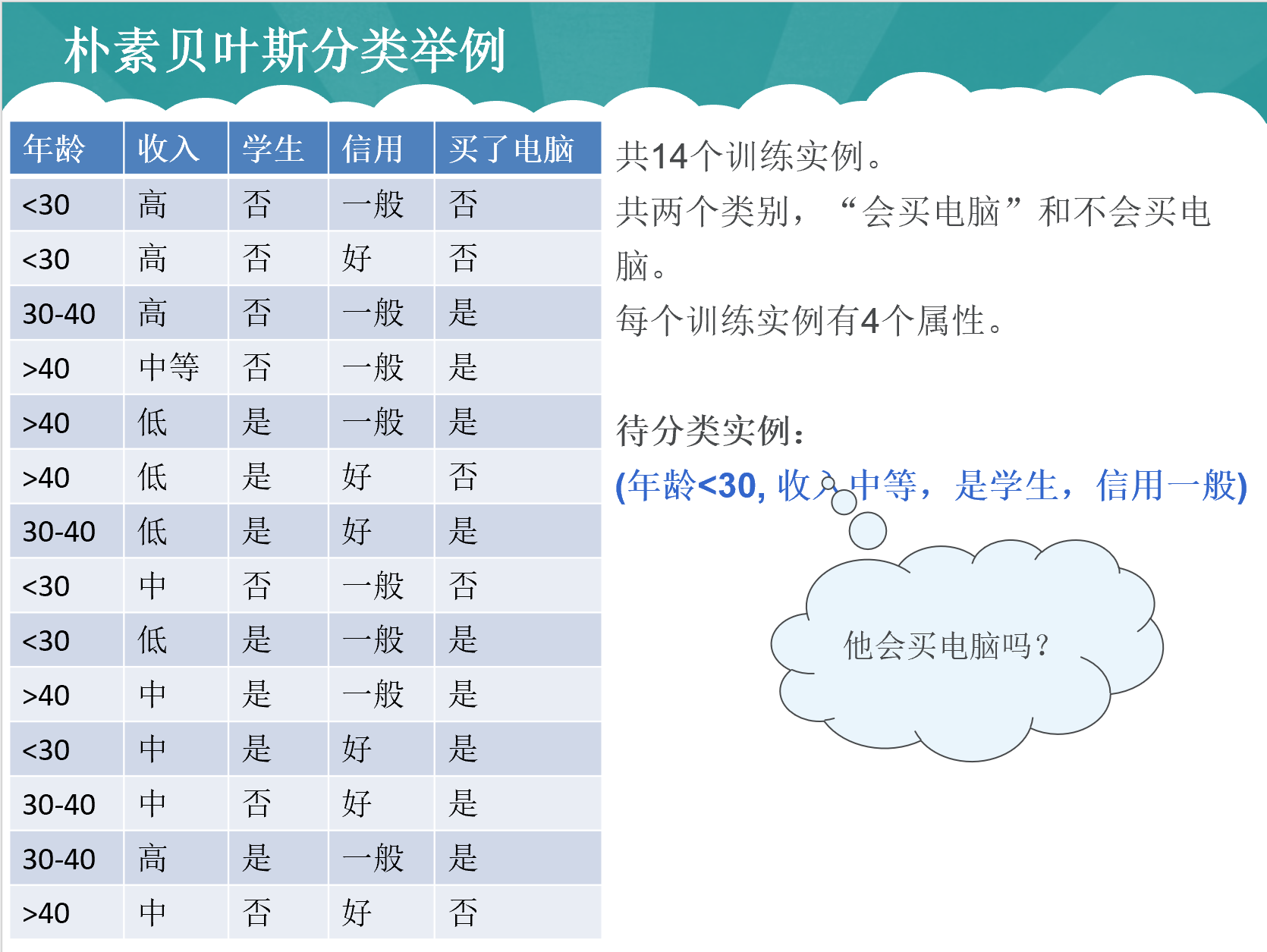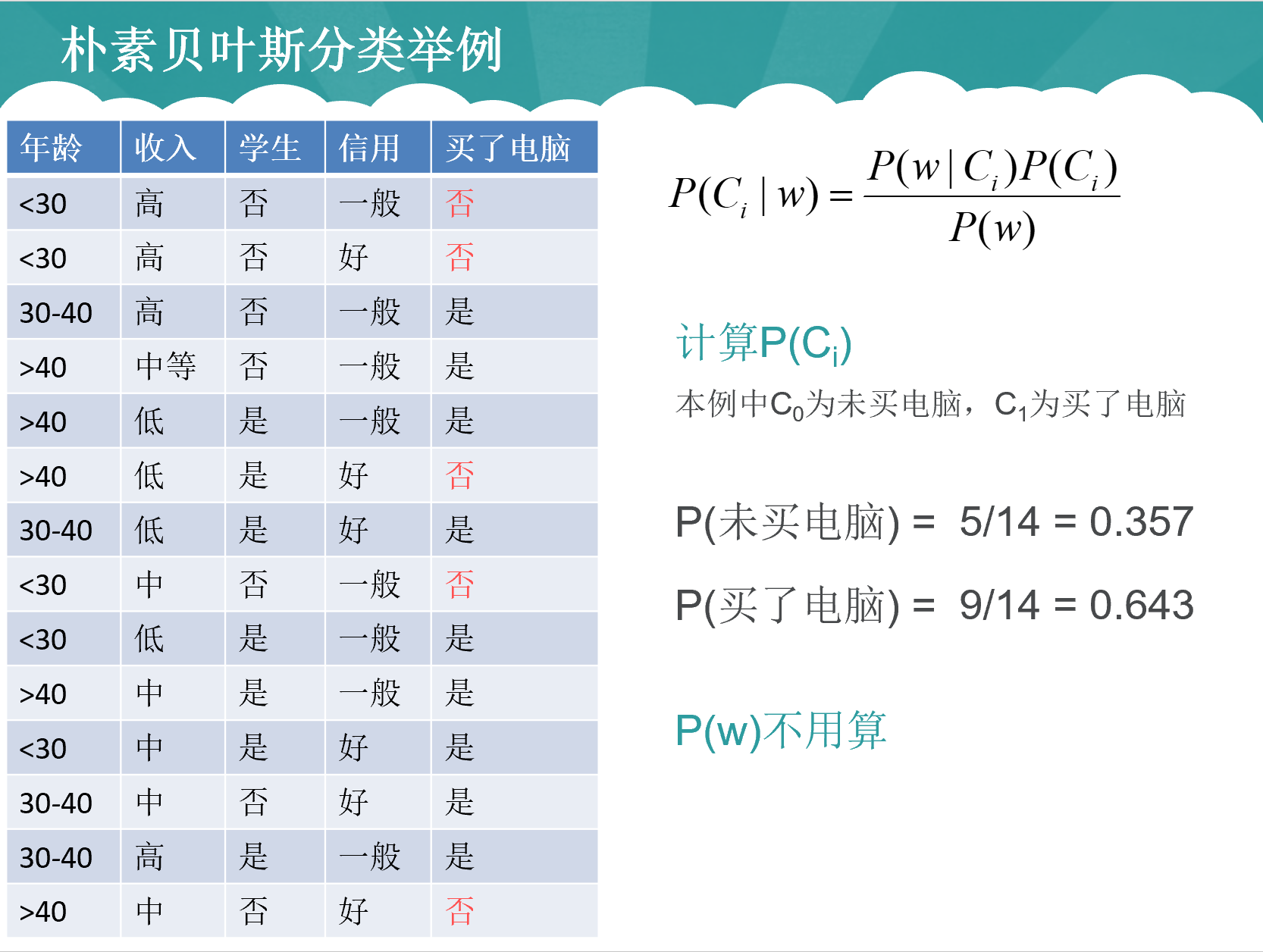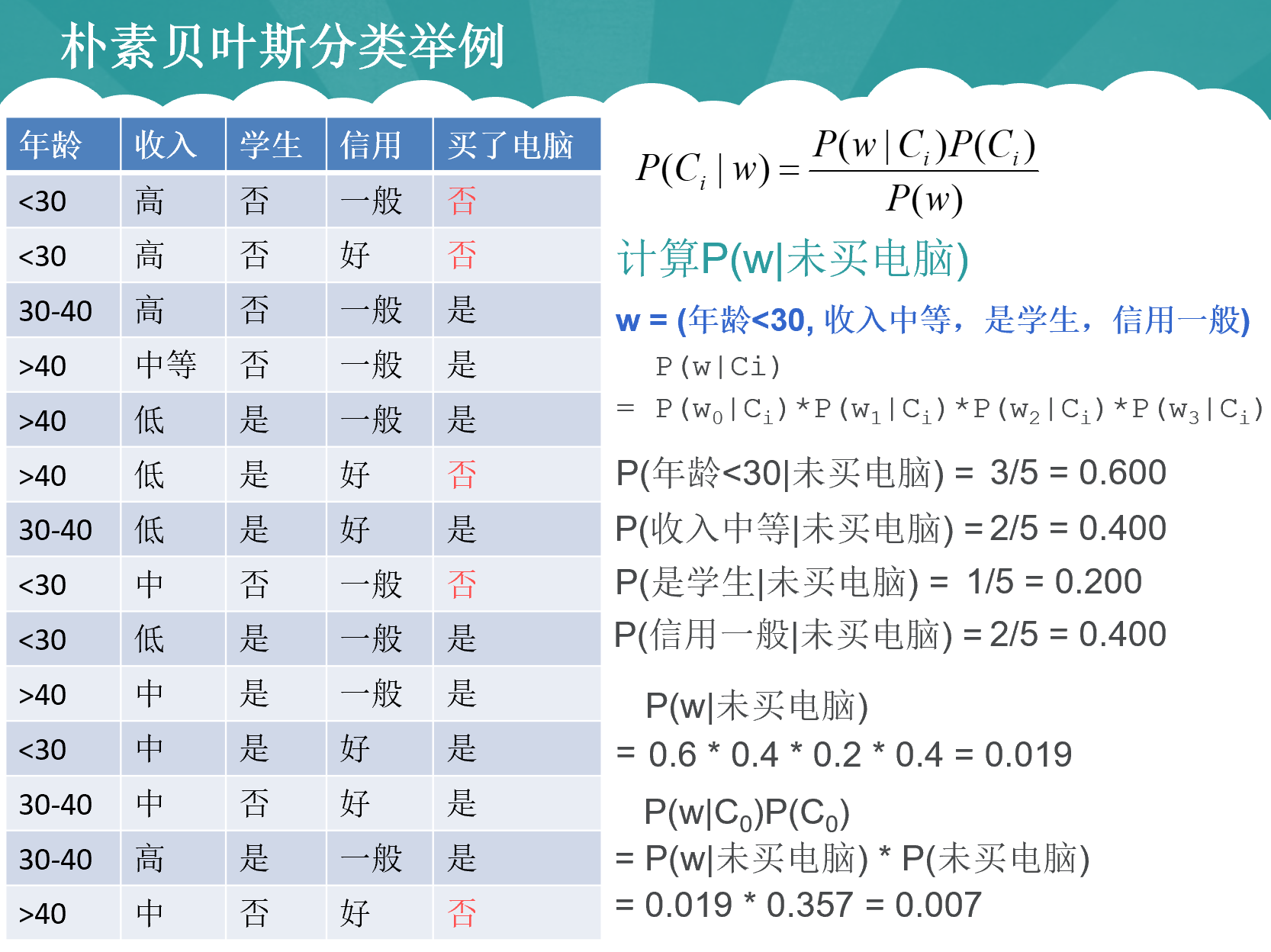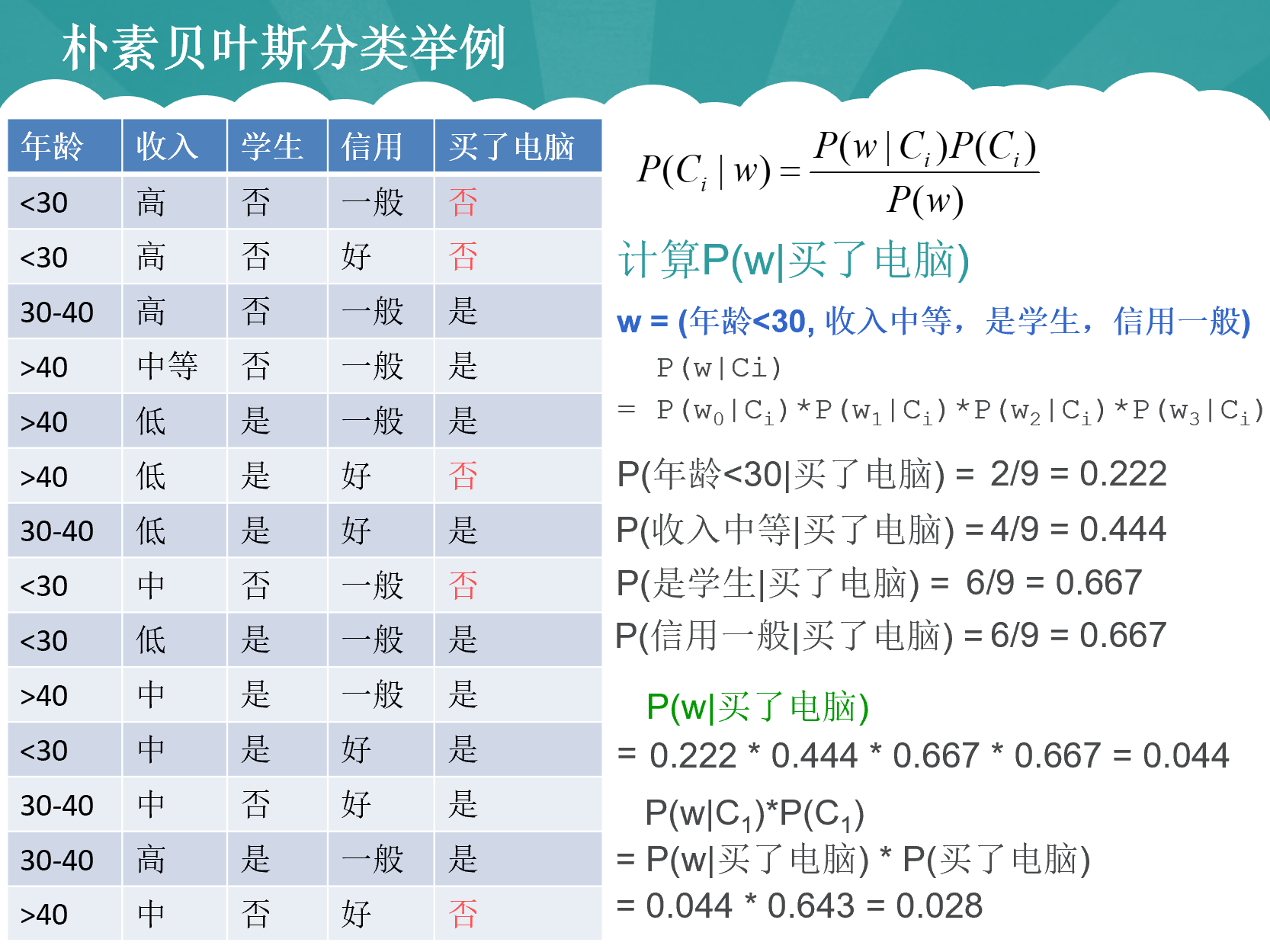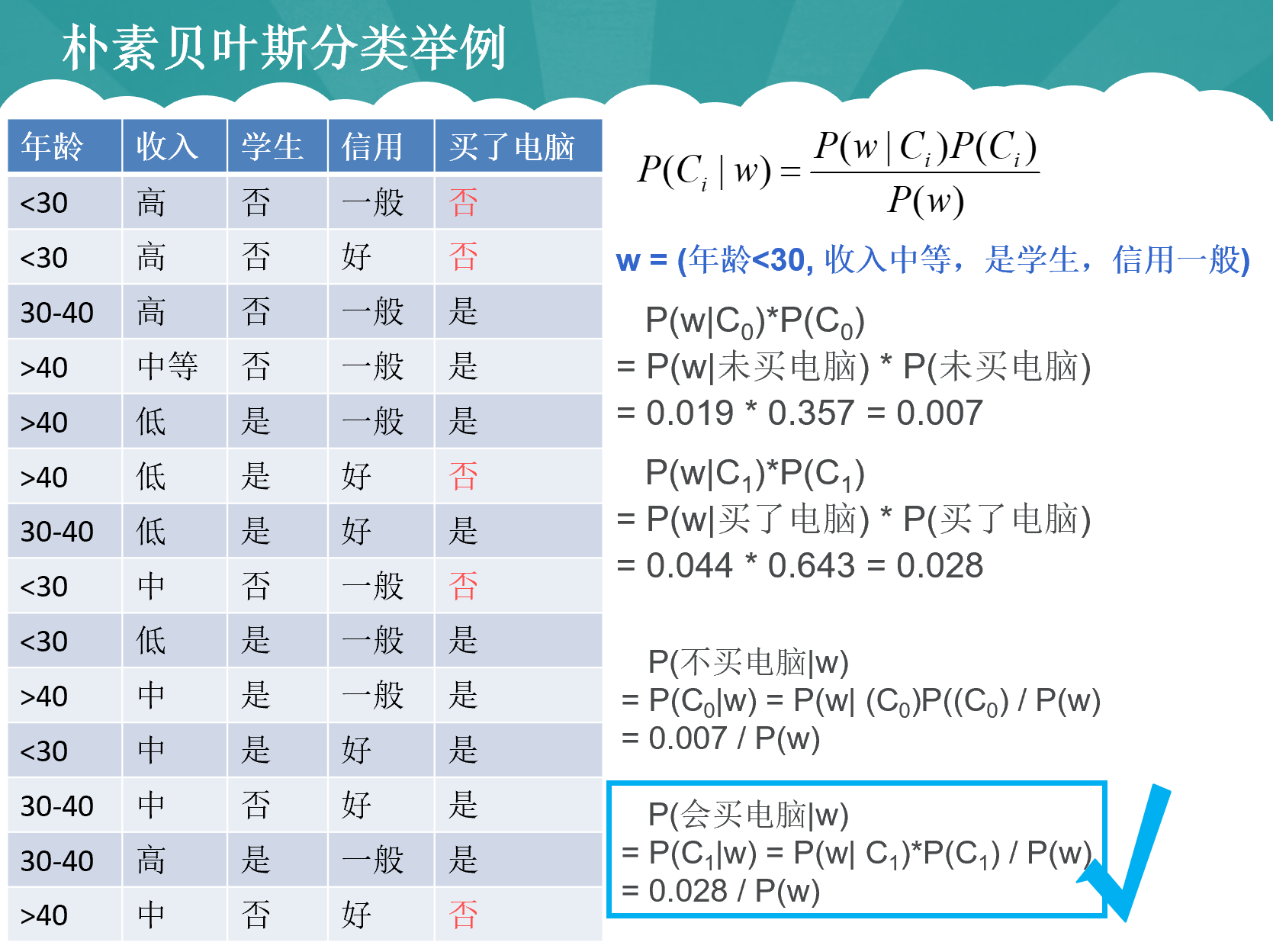展开全文• 本节主要介绍数据挖掘中常见的分类方法决策树和朴素贝叶斯算法。 决策树算法 决策树（Decision Tree，DT）分类法是一个简单且广泛使用的分类技术。 决策树是一个树状预测模型，它是由结点和有向边组成的层次结构。...
• 主要为大家详细介绍了Python实现朴素贝叶斯算法，具有一定的参考价值，感兴趣的小伙伴们可以参考一下
• 首先介绍以下贝叶斯公式：这个是什么意思呢？该如何用呢？相信大家有一定的概率知识基础的同学，对先验概率及条件概率都比较清楚，在这里简单的说一下这条公式的含义：1、首先代表的意思就是在B条件发生的情况下，Ai...

首先介绍以下贝叶斯公式：这个是什么意思呢？该如何用呢？相信大家有一定的概率知识基础的同学，对先验概率及条件概率都比较清楚，在这里简单的说一下这条公式的含义：

1、首先代表的意思就是在B条件发生的情况下，Ai发生的概率

2、这公式代表的意思就是，在所有A类的结果中，B发生的概率之和，也就是B的概率

3、所以在这个公式，表达的意思就是(B条件发生的情况下，Ai发生的概率=Ai与B同时发生的概率占整个B的概率的多少)

于是这个公式就可以转化为，(加上后面这个公式，是为了让同学们更好的理解，实际上的使用，一般只用第一个等式的公式)

4、因此，由于P(B)是固定得，所以发生得概率与分子相关，也就是分子越大，概率越高

仔细品了一下这个公式，也确实发现其很适合用在分类的算法中；对于这个贝叶斯公式来说，就像是求B属于Ai的概率，运用在分类中，就得先

求出B属于每一类中的可能，找出最大的

，这就是朴素贝叶斯的算法思路。

由此得到朴素贝叶斯分类算法流程：

1、利用贝叶斯公式，求出预测得结果属于每一种类别得可能

2、找出最大值

代码流程：

1、先将样本数据处理成字典，并统计每一类发生得个数(第一个函数)

2、将待预测得样本写入，并根据之前得样本数据，对分类结果进行求概率，得出最大概率得分类结果

#k代表分类的结果

#将数据处理成字典，用于保存样本数据中得类别数据存储情况

def chuli(data_1,k):

dict_1={}

col_num=data_1.columns.tolist()

col_num_1=col_num[1:-1]

all_number=len(data_1)

#all_result为分类的结果类型

all_result=dict(data_1.iloc[:,k-1].value_counts())

all_result_1=list(dict(data_1.iloc[:,k-1].value_counts()).keys())

#将每个结果写入

for result in all_result_1:

if result not in dict_1:

dict_1.setdefault(result,{})

for col in col_num_1:

if col not in dict_1[result]:

dict_1[result].setdefault(col,{})

all_result_2=data_1[col].value_counts().index.tolist()

for result_1 in all_result_2:

number_1=len(data_1.loc[(data_1[col_num[k-1]]==result) & (data_1[col]==result_1)])

if result_1 not in dict_1[result][col]:

dict_1[result][col].setdefault(result_1,number_1)

return dict_1,all_result

#对每待预测得结果进行贝叶斯公式得计算，并得出预测类别与概率

def jisuan(dict_1,dict_2,need_data_1):

new_col=need_data_1.columns.tolist()

new_list_5=[]

for i in range(len(need_data_1)):

all_result_2=dict_1.keys()

dict_4={}

for i1 in all_result_2:

new_list=[]

for col in range(len(new_col)):

number_1=dict_1[i1][new_col[col]][need_data_1.iloc[i,col]]/dict_2[i1]

new_list.append(number_1)

#print(dict_4)

all_sum=dict_2[i1]/sum(list(dict_2.values()))

for i2 in new_list:

all_sum=all_sum*i2

dict_4.setdefault(i1,all_sum)

#print(dict_4)

max_str=max(dict_4,key=dict_4.get)

max_value=dict_4[max_str]

new_list_5.append([max_str,max_value])

return new_list_5

这里放出一个我写过得案例：待预测数据：返回得两个存储字典：对待预测样本进行预测：根据自已手写得计算结果：P(No)*P(有房=是|No)*P(婚姻状况=已婚|No)=7/10*3/7*4/7=0.1714285714285714

而P(Yes)*P(有房=是|Yes)*P(婚姻状况=已婚|Yes)=3/10*0*0=0

所以得出结果为NO，概率为0.1714

展开全文• （一）贝叶斯原理中的几个概念 先验概率 通过经验来判断事情发生的概率，比如说某一种疾病的发病率是万分之一，就是先验概率。再比如南方的梅雨季是 6-7 月，就是通过往年的气候总结出来的经验，这个时候下雨的...python
• 朴素贝叶斯法是基于贝叶斯定理与特征条件独立性假设的分类方法。对于给定的训练集，首先基于特征条件独立假设学习输入输出的联合概率分布（朴素贝叶斯...学习朴素贝叶斯算法之前，我们先搞定下面这些基本概念和数学公式机器学习 python 算法 人工智能
• 朴素贝叶斯算法以及案例 大家好，我是W 这次给大家带来朴素贝叶斯算法，贝叶斯分类是一类分类算法的总称，其基础都是贝叶斯定理。要理解该算法就需要先理解其背后的概率知识，我会尽量详细地给大家讲解清楚。所以...机器学习 python
• 机器学习案例——基于朴素贝叶斯算法的文本分类（垃圾邮件过滤）的数据集，见本人的这篇博客！！！这个资源是本人搜集的支撑数据包！数据集 机器学习
• 朴素贝叶斯中的朴素是指假设各个特征之间相互独立，不会互相影响，所以称为朴素贝叶斯。正是因为这个假设，使得算法的模型简单且容易理解，虽然牺牲了一点准确性，但是如果模型训练的好，也能得到不错的分类效果。 ...
• 这篇文章我尽可能用直白的话语总结一下我们学习会上讲到的朴素贝叶斯分类算法，希望有利于他人理解。 1 分类问题综述 对于分类问题，其实谁都不会陌生，日常生活中我们每天都进行着分类过程。例如，当你看到...nlp
• sklearn应用朴素贝叶斯算法 通过两节知识的学习，相信你对朴素贝叶斯算法有了初步的掌握，本节将实际应用朴素贝叶斯算法，从实战中体会算法的精妙之处。 首先看下面一个简单应用案例： 简单应用案例 假设一个学校有 ...python 机器学习 sklearn
• 朴素贝叶斯法是基于贝叶斯定理与特征条件独立假设的分类方法。对于给定的训练数据集，首先基于特征条件独立假设学习输入输出的联合概率分布；然后基于此模型，对给定的输入x，利用贝叶斯定理求出后验概率最大的输出Y...机器学习
• 朴素贝叶斯算法原理讲解 1 算法抽象性解释 NaïveBayes算法，又叫朴素贝叶斯算法，是基于贝叶斯定理与特征条件独立假设的分类方法。 名称由来：朴素，即特征条ming件独立；贝叶斯：基于贝叶斯定理。所谓朴素，就是在...机器学习 算法
• 使用朴素贝叶斯进行训练(多项式模型) 23 mnb = MultinomialNB() 24 mnb.fit(x_train, y_train) 25 y_predict = mnb.predict(x_test) 26 27 # 获取预测结果 28 print (classification_report(y...
• 一、朴素贝叶斯算法介绍 朴素贝叶斯，之所以称为朴素，是因为其中引入了几个假设（不用担心，下文会提及）。而正因为这几个假设的引入，使得模型简单易理解，同时如果训练得当，往往能收获不错的分类效果，因此这...
• ## 朴素贝叶斯算法（带例题解释）

万次阅读 多人点赞 2020-02-23 23:18:04
朴素贝叶斯算法（Naive Bayesian algorithm) 是应用最为广泛的分类算法之一，在垃圾邮件分类等场景展露出了非常优秀的性能。 朴素贝叶斯公式来历 朴素贝叶斯，名字中的朴素二字就代表着该算法对概率事件做了很大的...数据挖掘 机器学习
• 1 朴素贝叶斯算法原理 贝叶斯分类是机器学习中应用极为广泛的分类算法之一。 朴素贝叶斯是贝叶斯模型当中最简单的一种，其算法核心为如下所示的贝叶斯公式。 其中P（A）为事件A发生的概率，P（B）为事件B...学习 python 数据挖掘 机器学习
• 朴素贝叶斯算法的原理： 1. 朴素贝叶斯法是典型的生成学习方法。 生成方法由训练数据学习联合概率分布P(X，Y)，然后求得后验概率分布P(Y|X)。具体来说，利用训练数据学习P(XY)和P(Y)的估计，得到联合概率分布：P(X，...机器学习 python...